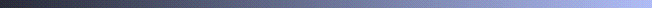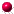## GSLIB Help Page: ProgramsCoordinate transformation:
• rotcoord 2-D coordinate rotationProbability distribution weighting, transformation, and smoothing:
• declus cell declustering
• nscore normal score transformation
• backtr back transformation from normal scores
• trans general distribution transformation
• histsmth smooth histogram / univariate distributionVariograms:
• gam variogram calculation of regular grid (use vargplt to plot results)
• gamv variogram calculation of scattered data (use vargplt to plot results)
• varmap variogram map / volume calculation (use pixelplt to plot results)
• vmodel creates a variogram from an analytical model that can be plotted with vargplt
• bigaus can be used to get the indicator variograms from a Gaussian or normal scores variogram
• The "variogram type" is specified by an integer code. The type of variogram model is specified by another integer code.Kriging:
• kb2d straightforward 2-D kriging
• kt3d flexible 3-D kriging
• cokb3d cokriging
• ik3d indicator kriging (use postik to postprocess results)Stochastic simulation:
• draw simple Monte Carlo stochastic simulation
• lusim LU matrix Gaussian simulation
• sgsim sequential Gaussian simulation
• gtsim truncated Gaussian simulation (uses the result of sgsim and proportion curves)
• sisim sequential indicator simulation including categorical and continuous and Markov-Bayes (program bicalib is used to process calibration data)
• pfsim probability field simulation
• ellipsim 3-D ellipsoid simulation
• anneal annealing-based post processing / simulation
• sasim annealing-based simulation and cosimulation
• postsim is used to post process a number of simulated realizationsPostScript plotting:
• histplt histogram and cumulative histogram
• probplt normal and lognnormal probability plot
• scatplt scatterplot
• qpplt Q-Q or P-P plot to compare two distributions
• locmap gray and color 2-D data location map
• pixelplt gray and color 2-D pixel map
• bivplt plot a smoothed bivariate probability distribution with the marginal distributions